﻿

## Newton's Method and Successive Approximation

The numerical solution of mathematical problems often involves making an estimate of the solution, and then using this estimated solution to refine a subsequent estimate of the solution. This is called successive approximation. A quite simple and elegant example of successive approximation is Newton’s Method for finding the roots. Numeric solutions of algebraic equations are estimates of the true roots while analytical solutions are exact. There is an error associated with the numerical solution estimate. How fast this error is reduced on each successive approximation is an important part of numerical solution algorithms.

Consider the expression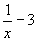To analytically find the root of this function (where it equals zero):

1. Set the function's expression equal to zero: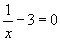2. Solve the resulting equation for x: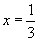To numerically find the root of this function using Newton's Method: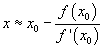1. Plot the function.
2. Choose an initial guess, x0, that is reasonably close to the root.
3. Compute the value of the derivative of the function at x0.
4. Compute the next approximation using Newton's formula.5. Repeat steps 3 and 4 using this more general form of Newton's Method until your approximation is as accurate as desired.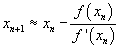Newton’s Method is used in the `fsolve` command in Maple and in the `fzero` function in MATLAB. However, for this module, it is useful to implement it yourself.

Newton's Method is important because modern computer processors use Newton’s Method to find reciprocals rather than implementing the long division algorithm in the hardware. Newton’s Method is actually faster to execute than long division!